#Function Repository Resource:

# NInverseFourierCoefficient

Find a numerical approximation for a function with a given Fourier exponential series

Contributed by: Wolfram Research
 ResourceFunction["NInverseFourierCoefficient"][expr,n,t] gives a numerical approximation to the function, evaluated at t, whose Fourier exponential series representation has coefficients given by expr, where expr is a function of n.

## Details and Options

The numerical approximation to the Fourier exponential series representation used by ResourceFunction["NInverseFourierCoefficient"] is by default defined to be NSum[expr-2πⅈnt,{n,-∞,}].
Different choices for the definition of the Fourier exponential series representation can be specified using the option FourierParameters.
With the setting FourierParameters{a,b}, the Fourier exponential series representation used by ResourceFunction["NInverseFourierCoefficient"] is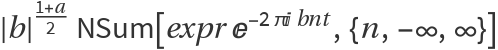.
The parameter b in the setting FourierParameters{a,b} must be numeric.
In addition to the option FourierParameters, ResourceFunction["NInverseFourierCoefficient"] can also accept the options available to NSum. These options are passed directly to NSum.

## Examples

### Basic Examples

Numerical approximation for a function with a given Fourier series:

 In:=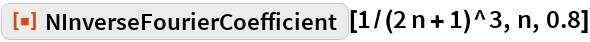Out=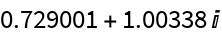Compare with the answer from symbolic evaluation:

 In:=Out=In:=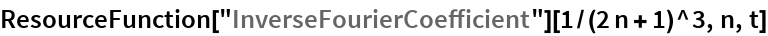Out=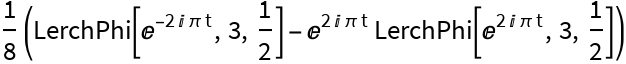In:=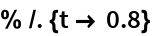Out=## Requirements

Wolfram Language 11.3 (March 2018) or above